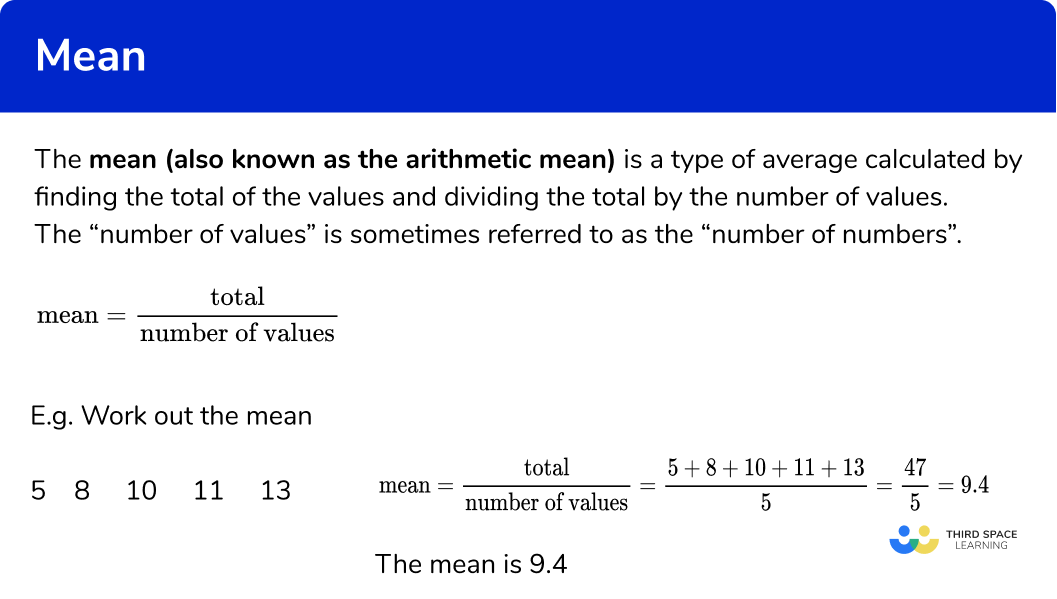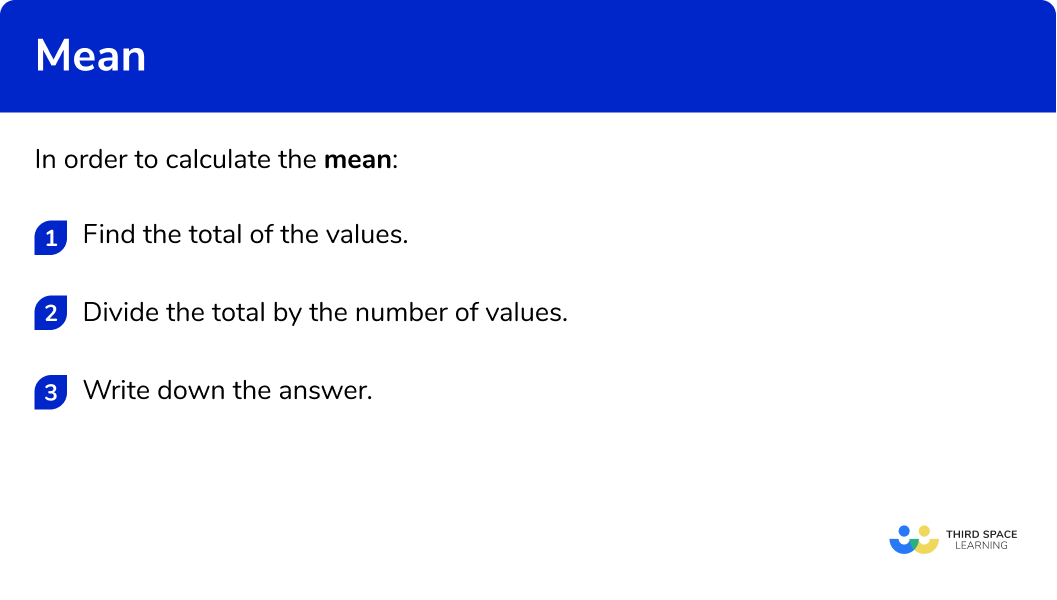# Mean

Here we will learn about the mean, including what the mean is and how to find the mean.

There are also mean worksheets based on Edexcel, AQA and OCR exam questions, along with further guidance on where to go next if you’re still stuck.

## What is the mean?

The mean is a type of average calculated by finding the total of the values and dividing the total by the number of values.

The “number of values” is sometimes referred to as the “number of numbers”.

\text{Mean}=\frac{\text{total}}{\text{number of values}}

E.g. Work out the mean

$\text{Mean}=\frac{\text{total}}{\text{number of values}}=\frac{5+8+10+11+13}{5}=\frac{47}{5}=9.4$

The mean is 9.4

The mean (also known as the arithmetic mean) is a measure of central tendency because it describes a set of numbers by identifying a central position within the data.

### What is the mean?## How to calculate the mean

In order to calculate the mean:

1. Find the total of the values.
2. Divide the total by the number of values.

### How to calculate the mean## Related lessons on mean, median, mode

Mean is part of our series of lessons to support revision on mean, median, mode. You may find it helpful to start with the main mean, median, mode lesson for a summary of what to expect, or use the step by step guides below for further detail on individual topics. Other lessons in this series include:

## Mean maths examples

### Example 1: finding the mean

Calculate the mean value of this list of numbers:

2 7 9 10 12

1. Find the total of the values.

Add up all the values in the list

\text{Total}=2+7+9+10+12=40

2Divide the total by the number of values.

There are 5 values in the data set. We need to divide the total by 5 .

\text{Mean}=\frac{\text{total}}{\text{number of values}}=\frac{40}{5}=8

If your answer is a decimal, you may need to round your answer to a given number of decimal places or significant figures.

Here the mean is an integer, so there is no need to round.

The mean is 8

### Example 2: finding the mean

Calculate the mean value of this set of numbers.

13 16 17 17 18 20

Find the total of the values.

Divide the total by the number of values.

### Example 3: finding the mean

Calculate the mean value of this set of data. Give your answer to 1 decimal place.

11 13 14 15 19 20 22

Find the total of the values.

Divide the total by the number of values.

### Example 4: finding the mean

Calculate the mean value of this list of numbers.

101 102 105 106 108

Find the total of the values.

Divide the total by the number of values.

## How to solve a problem involving the mean

In order to solve a problem involving the mean:

1. Use the mean and number of values to find the total.
2. Read the question carefully to work the next step.

## Problem solving involving mean examples

### Example 5: problem solving

The mean of 4 values is 10 .

Here are 3 of the values:

6 9 12

Find the 4 th value.

Use the mean and number of values to find the total.

Read the question carefully to work the next step.

### Example 6: problem solving

The mean of 5 values is 14 .

Here are 4 of the values:

5 11 13 19

Find the 5 th value:

Use the mean and number of values to find the total.

Read the question carefully to work the next step.

### Example 7: problem solving involving 2 means (HIGHER)

Data Set A has 6 values and a mean of 10 .

Data Set B has 4 values and a mean of 8 .

Find the mean of all 10 values.

Use the mean and number of values to find the total.

Read the question carefully to work the next step.

### Example 8: problem solving involving 2 means (HIGHER)

Data Set A has 4 values and a mean of 8 .

Data Set B has 7 values and a mean of 6 .

Find the mean of the all 11 values. Give your answer correct to 2 decimal places.

Use the mean and number of values to find the total.

Read the question carefully to work the next step.

### Common misconceptions

• Check which average you are being asked for

Check if you have been asked for the median, mode, or mean average.

• The mean average can be a decimal

The mean does not have to be a whole number. It can be a decimal or a fraction. It may be a decimal which needs rounding.

### Practice mean maths questions

1. Find the mean of this list of values:
5 7 8 8 9

77.47.58\text{Mean}= \frac{\text{total}}{\text{number of values}}\\ \text{Mean}=\frac{5+7+8+8+9}{5}\\ \text{Mean}= \frac{37}{5}\\ \text{Mean}=7.4

2. Find the mean of this list of values:
3 5 6 9

5.75.755.85.9\text{Mean}= \frac{\text{total}}{\text{number of values}}\\ \text{Mean}=\frac{3+5+6+9}{4}\\ \text{Mean}= \frac{23}{4}\\ \text{Mean}=5.75

3. Find the mean of this list of values. Give your answer to 3 significant figures:
7 8 9 10 10 11

9.169.5109.17\text{Mean}= \frac{\text{total}}{\text{number of values}}\\ \text{Mean}=\frac{7+8+9+10+10+11}{6}\\ \text{Mean}= \frac{55}{6}\\ \text{Mean}=9.16666…\\ \text{Mean}=9.17 \ \text{(to 3 sf)}

4. Find the mean of this list of values. Give your answer to 3 significant figures:
17 18 18 19 20 21 25

181919.819.7\text{Mean}= \frac{\text{total}}{\text{number of values}}\\ \text{Mean}=\frac{17+18+18+19+20+21+25}{7}\\ \text{Mean}= \frac{55}{7}\\ \text{Mean}=19.7142…\\ \text{Mean}=19.7\ \text{(to 3 sf)}

5. The mean of 4 numbers is 9 .
Here are 3 of the numbers
6 8 15
What is the 4 th number?

7568The total of 4 numbers is:
\text{Total of 4 values}=\text{mean} \times \text{number of values}=9\times 4=36

The total of 3 numbers is: 6+8+9=29

The difference between the totals is: 36-29=7

This is the missing number.

6. The mean of 6 numbers is 12 .
Here are 5 of the numbers
7 9 11 13 18
What is the 6 th number?

12141315The total of 4 numbers is:
\text{Total of 4 values}=\text{mean} \times \text{number of values}=12\times 6=72

The total of 5 numbers is: 7+9+11+13+18=58

The difference between the totals is: 72-58=14

This is the missing number.

### Mean maths GCSE questions

1. Here is a list of numbers:Work out the mean of the numbers in this list.

(2 marks)

\frac{1+3+4+6+6}{5}=\frac{20}{5}
For adding the numbers and dividing by 5

(1)

=5

(1)

2. Here are the ages of people in a familyWork out the mean of the ages in this family.

(2 marks)

\frac{15+15+19+20+54+59}{6}=\frac{182}{6}
For adding the numbers and dividing by 6

(1)

\frac{182}{6}=30.333…=30.3 \ \text{(to 3 sf)}

(1)

3. Dev has 5 tests and achieves an average of 61% .
Here are 4 of the results.Find the missing result.

(2 marks)

5\times 61-(48+59+65+73)
For subtracting the total of 4 results from the total of 5 results

(1)

305-245=60

(1)

4. HIGHER
5 red bricks have a mean weight of 10 kg .
3 blue bricks have a mean weight of 6 kg .
Find the mean of the 8 bricks.

(3 marks)

5\times 10=50

3\times6=18

For finding the total weights of the red bricks and the blue bricks

(1)

\frac{50+18}{8}=\frac{68}{8}
For dividing the total weight of 8 bricks by 8

(1)

=8.5 \ \text{kg}

(1)

## Learning checklist

You have now learned how to:

• Calculate the mean
• Solve problems involving the mean
• Higher – calculate the combined mean

## Still stuck?

Prepare your KS4 students for maths GCSEs success with Third Space Learning. Weekly online one to one GCSE maths revision lessons delivered by expert maths tutors.

Find out more about our GCSE maths tuition programme.